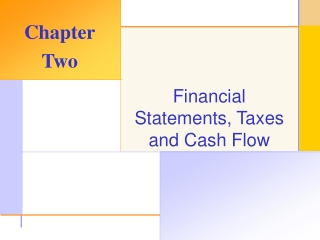# Financial Statements, Taxes and Cash Flow - PowerPoint PPT PresentationDownload PresentationFinancial Statements, Taxes and Cash Flow

Financial Statements, Taxes and Cash Flow
Download Presentation## Financial Statements, Taxes and Cash Flow

- - - - - - - - - - - - - - - - - - - - - - - - - - - E N D - - - - - - - - - - - - - - - - - - - - - - - - - - -
##### Presentation Transcript

1. Chapter Two Financial Statements, Taxes and Cash Flow

2. Key Concepts and Skills • Know the difference between book value and market value • Know the difference between accounting income and cash flow • Know how to determine a firm’s cash flow from its financial statements

3. Chapter Outline • The Balance Sheet • The Income Statement • Cash Flow

4. Balance Sheet • The balance sheet is a snapshot of the firm’s assets and liabilities at a given point in time • Assets are listed in order of liquidity • Ease of conversion to cash • Without significant loss of value • Balance Sheet Identity • Assets = Liabilities + Stockholders’ Equity

5. The Balance Sheet - Figure 2.1

6. Net Working Capital and Liquidity • Net Working Capital • Current Assets – Current Liabilities • Positive when the cash that will be received over the next 12 months exceeds the cash that will be paid out • Usually positive in a healthy firm • Liquidity • Ability to convert to cash quickly without a significant loss in value • Liquid firms are less likely to experience financial distress • But, liquid assets earn a lower return • Trade to find balance between liquid and illiquid assets

7. US Corporation Balance Sheet – Table 2.1

8. Market Vs. Book Value • The balance sheet provides the book value of the assets, liabilities and equity. • Market value is the price at which the assets, liabilities or equity can actually be bought or sold. • Market value and book value are often very different. Why? • Which is more important to the decision-making process?

9. Example 2.2 Klingon Corporation

10. Income Statement • The income statement is more like a video of the firm’s operations for a specified period of time. • You generally report revenues first and then deduct any expenses for the period • Matching principle – GAAP say to show revenue when it accrues and match the expenses required to generate the revenue

11. US Corporation Income Statement – Table 2.2

12. The Concept of Cash Flow • Cash flow is one of the most important pieces of information that a financial manager can derive from financial statements • The statement of cash flows does not provide us with the same information that we are looking at here • We will look at how cash is generated from utilizing assets and how it is paid to those that finance the purchase of the assets

13. Cash Flow From Assets • Cash Flow From Assets (CFFA) = Cash Flow to Creditors + Cash Flow to Stockholders • Cash Flow From Assets = Operating Cash Flow – Net Capital Spending – Changes in NWC

14. Example: US Corporation • OCF (I/S) = EBIT + depreciation – taxes = \$547 • NCS ( B/S and I/S) = ending net fixed assets – beginning net fixed assets + depreciation = \$130 • Changes in NWC (B/S) = ending NWC – beginning NWC = \$330 • CFFA = 547 – 130 – 330 = \$87 • CF to Creditors (B/S and I/S) = interest paid – net new borrowing = \$24 • CF to Stockholders (B/S and I/S) = dividends paid – net new equity raised = \$63 • CFFA = 24 + 63 = \$87

15. Cash Flow Summary Table 2.5

16. Example: Balance Sheet and IncomeStatement Information • Current Accounts • 1998: CA = 1500; CL = 1300 • 1999: CA = 2000; CL = 1700 • Fixed Assets and Depreciation • 1998: NFA = 3000; 1999: NFA = 4000 • Depreciation expense = 300 • LT Liabilities and Equity • 1998: LTD = 2200; Common Equity = 500; RE = 500 • 1999: LTD = 2800; Common Equity = 750; RE = 750 • Income Statement Information • EBIT = 2700; Interest Expense = 200; Taxes = 1000; Dividends = 1250

17. Example: Cash Flows • OCF = 2700 + 300 – 1000 = 2000 • NCS = 4000 – 3000 + 300 = 1300 • Changes in NWC = (2000 – 1700) – (1500 – 1300) = 100 • CFFA = 2000 – 1300 – 100 = 600 • CF to Creditors = 200 – (2800 – 2200) = -400 • CF to Stockholders = 1250 – (750 – 500) = 1000 • CFFA = -400 + 1000 = 600 • The CF identity holds.

18. Quick Quiz • What is the difference between book value and market value? Which should we use for decision making purposes? • What is the difference between accounting income and cash flow? Which do we need to use when making decisions? • What is the difference between average and marginal tax rates? Which should we use when making financial decisions? • How do we determine a firm’s cash flows? What are the equations and where do we find the information?